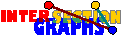# Betti numbers of intersection graphs

Under certain conditions, the topology of the union of the hyperedges is to some extend reflected in the structure of its intersection graph. This is the reason why the octahedron is no intersection graph of rectangles in the plane, but of unit disks, for instance.

When speaking of the topology of a graph, the 1-dimensional simplicial complex is not relevant for our purposes, instead let us define the simplicial complex G^ of a graph to be the complex whose simplices are the vertex sets of all complete subgraphs of G. We compare the topology of this complex with the topology of the union of all sets in the geometric case. In the discrete case, we define the complex of a hypergraph H=(A,(Sx/xV)) to have all subsets of the hyperedges as simplices.

The connection between the topologies is not too astonishing, since there is some connection between the union of these geometric sets, respectively , to the nerve of the hypergraph, which is just H*°. This follows by classical topological theorems. On the other hand, G^ is just a subcomplex of this nerve, and sometimes they even coincide.H*°=G^ if and only if H obeys the Helly property.

We distinguish these topological spaces by means of their (modulo 2) Betti numbers, i.e. ranks of the modulo 2 homology groups. You may think of0 counting the connected components of the space,1 counting the number of independent `tunnels' through the space,2 counting the number of independent `Swiss cheese holes', and so on.

Certainly these numbers are interesting, since, by the Euler-Poincare- Formula, they are connected to the numbers at(G) of t-element complete subgraphs of the graph G: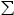t (-1)tt(G^) =t (-1)t at(G)

Let us illustrate these concepts: The complexes of the octahedron graphs are homotopic to the spheres, we havei (Ok^) =1 just for i=0,n-1, and =0 for all other i.

### Geometric models

A system of subsets of Rd has the Leray-property if every nonempty intersection is homologically trivial (i.e. has0=1, but 0=1 =2 =...). For many properties P, the Leray-property is automatically fulfilled for every system of P-sets. Examples are straight lines, or unit-disks, or boxes.

The key for homology for such families lies in the following classical result:[L45]: Nerve and union are homological equivalent for every family of subsets of Rd obeying the Leray-property.Let G be the intersection graph of a family (Sx/ xV) of subsets of Rd obeying both the Helly- and the Leray-property. Then G^ andSx are homologically equivalent. In particular 0=d(G^) =d+1(G^) = ... and Od+1 is not an induced subgraph of G.
 Proof: The first statement follows by Leray`s Theorem and the lemma above. The second follows immediately since higher homology groups of subsets of Rd vanish.

Therefore, for every property P where the Helly- and Leray-properties are both true for every family of P-sets, computing maximum cliques in G(P) is polynomial. An example is the class of intersection graphs of boxes. Another one subtrees of trees:0=1(G^) =2(G^) = ... for every chordal graph G.
 Proof: Subtrees of some tree obey both the Helly- and the Leray-property. By the corollary above, G^ is homologically equivalent to some substructure of the tree.
What happens if either Helly or Leray property are violated? See here for examples and problems.

### Discrete models

For discrete models, we only need the Helly-property. The Leray-property is, in a sense, automatically fulfilled.[D52]: For every hypergraph H, H° and H*° are homological equivalent.Let G be the intersection graph of a Helly-hypergraph (A,(Sx/ xV)). Then G^ and H*° are homologically equivalent. In particular 0=r(G^) =r+1(G^) =..., where r = max |Sx|, and Od+1 is not an induced subgraph of G.

Without the Helly property, not too much is known. Let us concentrate on intersection graphs of simple k-uniform hypergraphs again. For k=2 we have an almost complete picture:[P91]: For every graph G,1(G^) =1(L(G)^,2(G^)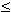2(L(G)^, andi(L(G)^) = 0 for all i >2.

Unfortunately nothing is known for higher k. Since large enough octahedron graphs are forbidden, we might ask:For which k>1 is there some f(k) such that every intersection graph G of a simple k-uniform hypergraph obeysi(G^)=0 for every i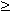f(k)?

Certainly f(2)=3, and also f(k){2k-1 choose k}, if existing at all.

We close by the following connection between intersection graphs of dual hypergraphs, which at least partially answers the first half of that problem above:[P***]: For every hypergraph H,1(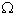(H)^) =1((H*)^)

Thus concerning the "A eats B" example, if the competition graph is an interval graph, then the first Betti number of the complex of the resource graph must be 0.

Back to the start page for intersection graphs.

Erich Prisner
made on January 12, 1999, last changed on February 5, 1999.